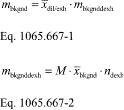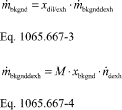# Title 40

## SECTION 1065.667

### 1065.667 Dilution air background emission correction.

§ 1065.667 Dilution air background emission correction.

(a) To determine the mass of background emissions to subtract from a diluted exhaust sample, first determine the total flow of dilution air, ndil, over the test interval. This may be a measured quantity or a calculated quantity. Multiply the total flow of dilution air by the mean mole fraction (i.e., concentration) of a background emission. This may be a time-weighted mean or a flow-weighted mean (e.g., a proportionally sampled background). Finally, multiply by the molar mass, M, of the associated gaseous emission constituent. The product of ndil and the mean molar concentration of a background emission and its molar mass, M, is the total background emission mass, m. In the case of PM, where the mean PM concentration is already in units of mass per mole of sample, M PM, multiply it by the total amount of dilution air flow, and the result is the total background mass of PM, mPM. Subtract total background mass from total mass to correct for background emissions.

(b) You may determine the total flow of dilution air by a direct flow measurement.

(c) You may determine the total flow of dilution air by subtracting the calculated raw exhaust molar flow as described in § 1065.655(g) from the measured dilute exhaust flow. This may be done by totaling continuous calculations or by using batch results.

(d) You may determine the total flow of dilution air from the measured dilute exhaust flow and a chemical balance of the fuel, intake air, and dilute exhaust as described in § 1065.655. For this option, the molar flow of dilution air is calculated by multiplying the dilute exhaust flow by the mole fraction of dilution gas to dilute exhaust, xdil/exh, from the dilute chemical balance. This may be done by totaling continuous calculations or by using batch results. For example, to use batch results, the total flow of dilution air is calculated by multiplying the total flow of diluted exhaust, ndexh, by the flow-weighted mean mole fraction of dilution air in diluted exhaust, x dil/exh. Calculate x dil/exh using flow-weighted mean concentrations of emissions in the chemical balance, as described in § 1065.655. The chemical balance in § 1065.655 assumes that your engine operates stoichiometrically, even if it is a lean-burn engine, such as a compression-ignition engine. Note that for lean-burn engines this assumption could result in an error in emission calculations. This error could occur because the chemical balance in § 1065.655 treats excess air passing through a lean-burn engine as if it was dilution air. If an emission concentration expected at the standard is about 100 times its dilution air background concentration, this error is negligible. However, if an emission concentration expected at the standard is similar to its background concentration, this error could be significant. If this error might affect your ability to show that your engines comply with applicable standards, we recommend that you either determine the total flow of dilution air using one of the more accurate methods in paragraph (b) or (c) of this section, or remove background emissions from dilution air by HEPA filtration, chemical adsorption, or catalytic scrubbing. You might also consider using a partial-flow dilution technique such as a bag mini-diluter, which uses purified air as the dilution air.

(e) The following is an example of using the flow-weighted mean fraction of dilution air in diluted exhaust, x dil/exh, and the total mass of background emissions calculated using the total flow of diluted exhaust, ndexh, as described in § 1065.650(c):Example: MNOx = 46.0055 g/mol x bkgnd = 0.05 µmol/mol = 0.05⋅10−6 mol/mol ndexh = 23280.5 mol x dil/exh = 0.843 mol/mol mbkgndNOxdexh = 46.0055⋅0.05⋅10−6⋅23280.5 mbkgndNOxdexh = 0.0536 g mbkgndNOx = 0.843 ⋅ 0.0536 mbkgndNOx = 0.0452 g

(f) The following is an example of using the fraction of dilution air in diluted exhaust, xdil/exh, and the mass rate of background emissions calculated using the flow rate of diluted exhaust, n dexh, as described in § 1065.650(c):Example: MNOx = 46.0055 g/mol xbkgnd = 0.05 µmol/mol = 0.05⋅10−6 mol/mol n dexh = 23280.5 mol/s xdil/exh = 0.843 mol/mol m bkgndNOxdexh = 46.0055⋅0.05⋅10−6⋅23280.5 m bkgndNOxdexh = 0.0536 g/hr m bkgndNOx = 0.843 ⋅ 0.0536 m bkgndNOx = 0.0452 g/hr [76 FR 57465, Sept. 15, 2011, as amended at 81 FR 74188, Oct. 25, 2016]